﻿ 杠杆率监管能有效降低银行体系系统性风险吗？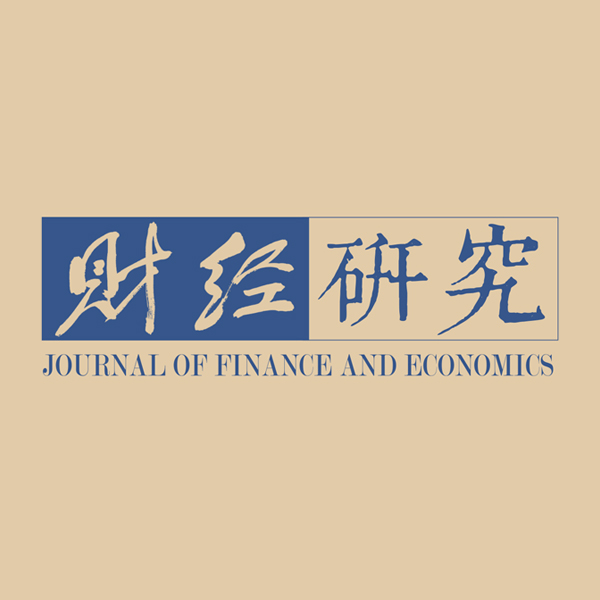《财经研究》
2020第46卷第2期

1. 中国人民银行成都分行 金融研究处，四川 成都 610000;
2. 成都银行 风险管理部，四川 成都 610000

Does Leverage Regulation Effectively Reduce the Systemic Risk of the Banking System? Simulation Analysis Based on the Endogenous Network Model
Lu Lu1,
1. Financial Research Department，Chengdu Branch of the People’s Bank of China，Chengdu 610000，China;
2. Risk Management Department，Bank of Chengdu，Chengdu 610000，China
Summary: Deleveraging of the banking system is an important task of financial supply-side structural reform in China, and also a key measure to prevent and resolve financial risks in recent years. With reference to Basel III, China Banking Regulatory Commission formally introduced the leverage regulation indicator in 2011. According to the official data, the leverage ratio of China’s major commercial banks has been higher than the minimum regulatory requirement of 4%, but it is also an indisputable fact that the risk of China’s banking system continues to gather after the financial crisis. So does leverage regulation effectively reduce the systemic risk of the banking system? The answer to this question is related to the effectiveness of the leverage as a macro prudential regulatory tool. However, limited by the lack of empirical data, the complexity of the economic and financial system and other factors, the current literature on the effectiveness of leverage as a macro prudential regulatory tool is rare.　　Therefore, based on the model of Bluhm, et al. (2014), this paper constructs an endogenous network model with leverage constraints, and simulates the risk contagion mechanism and effect under leverage ratio constraints. It is found that in the normal period, leverage regulation will bring the effect of increasing the proportion of risk assets of some banks and decreasing the average degree of network connection between banks. In the crisis period, leverage regulation will increase the number of initial bank failures under exogenous shocks, but will reduce the depth and breadth of risk contagion. On the whole, it is true that the minimum leverage requirement of 4% will lead to a more stable banking system, but the excessive leverage requirement may cause the rise of the systemic risk. In order to maintain the stability of the banking system to the greatest extent, we can implement counter-cyclical and differentiated leverage regulation strategies for banks. At the same time, timely liquidity assistance to the market and improving the loan asset recovery rate of defaulting banks during the crisis will help to enhance the financial stability effect brought by leverage regulation.　　The possible marginal contribution of this paper is as follows: Firstly, compared with the existing litera- ture on the effectiveness evaluation of macro prudential tools, based on the analysis of the endogenous network model, this paper opens the “black box” of leverage regulatory tools affecting the stability of the banking system, and explains the effect and mechanism of leverage regulation. Secondly, the existing endogenous network model usually fails to consider the impact of the introduction of leverage regulation conditions on the stability of the banking system in normal and crisis periods, which is emphasized in this paper. Thirdly, the study of this paper has strong policy significance. The comparative analysis of the regulatory effect of leve- rage under different market parameter combinations provides theoretical support for regulators to formulate more effective leverage regulatory strategies.
Key words: leverage regulation    endogenous network model    systemic risk

（一）银行行为

 ${\max _{{l^i}, \; n{l^i},\; b{l^i},\; b{b^i}}}\;\;E({\pi ^i}) = b{l^i} \times {r^{rf}} + \frac{{{r^i}}}{p} \times n{l^i} - b{b^i} \times {r^{rf}} \times \frac{1}{{1 - \varepsilon \times p{d^i}}}$ (1)

$b{l^i} =\sum_{j = 1}^Nb{l^{ij}},b{b^i} = \sum_ {j = 1}^Nb{b^{ij}}$
j≠i）。rrfp分别为银行间市场的无风险利率和非流动性资产的市场价格，基于下文的集中试错过程（centralized tâtonnement process）得到。pd i为其他经济主体长期观察到的银行i的期望违约概率，基于迭代算法得到。银行i的借款成本由银行间市场无风险利率rrf和风险溢价补偿因子（1−ε×pd i的倒数）共同决定，其他经济主体向银行i借款时会考虑其违约风险。银行违约损失率ε和非流动性资产收益ri均为外生变量。根据《巴塞尔协议Ⅲ》的要求，银行持有的现金不得低于存款的一定比例α，即：

 ${l^i} \geqslant a \times {d^i}$ (2)

 $\frac{{{l^i} + p \times n{l^i} + b{l^i} + m{l^i} + {d^i} \times h - {d^i} - b{b^i}}}{{{\chi _1} \times p \times n{l^i} + {\chi _2} \times b{l^i} + {\chi _3} \times m{l^i}}} \geqslant {\gamma ^ * } + \tau$ (3)
 $\frac{{{l^i} + p \times n{l^i} + b{l^i} + m{l^i} + {d^i} \times h - {d^i} - b{b^i}}}{{{l^i} + p \times n{l^i} + b{l^i} + m{l^i} + {d^i} \times h}} \geqslant le{v^ * }$ (4)

 ${l^i} + p \times n{l^i} + b{l^i} + {d^i} \times h + m{l^i} - b{b^i} = {d^i} + {e^i}$ (5)
 $n{l^i} \geqslant 0, \; {l^i} \geqslant 0, \; b{l^i} \geqslant 0, \; b{b^i} \geqslant 0$ (6)

（二）银行间市场均衡

 $D{E_i} = \sum\limits_{j \in M(i)} {{a_{ij}}}$ (7)
 $DE_i^{out} = \sum\limits_{j \in {M^{out}}(i)} {{a_{ij}}} , \; \; {\rm{ }}DE_i^{in} = \sum\limits_{j \in {M^{in}}(i)} {{a_{ij}}}$ (8)

 ${C_i} = \frac{2}{{D{E_i}(D{E_i} - 1)}}\sum\limits_{j,\;y} {{a_{ij}}{a_{iy}}{a_{jy}}}$ (9)

 ${C_B}(\nu ) = \sum\limits_{i \ne v \ne j \in V} {\frac{{{\sigma _{ij}}(\nu )}}{{{\sigma _{ij}}}}}$ (10)

（三）非流动性资产市场均衡

$s_{1}^{i}$
，则有：

 ${\gamma ^ * } = \frac{{p \times \left( {n{l^i} - s_1^i} \right) + {l^i} + b{l^i} + m{l^i} + {d^i} \times h + p \times s_1^i - b{b^i} - {d^i}}}{{{\chi _1} \times p \times (n{l^i} - s_1^i) + {\chi _2} \times b{l^i}{\rm{ + }}{\chi _3} \times m{l^i}}}$ (11)
 $s_1^i = \frac{{n{l^i} \times p \times ({\chi _1} \times {\gamma ^ * } - 1) + b{l^i} \times ({\chi _2} \times {\gamma ^ * } - 1) + m{l^i} \times ({\chi _3} \times {\gamma ^ * } - 1) + {d^i} \times \left( {1{\rm{ - }}h} \right) + b{b^i} - {l^i}}}{{{\chi _1} \times {\gamma ^ * } \times p}}$ (12)

levi<lev*时，为了满足杠杆率监管要求，银行将依次减少流动性资产和卖出非流动性资产以偿还部分存款债务。设所需减少的债务为Δdi（Δdi

$\leqslant$
di），则有：

 $1{\rm{ - }}\frac{{{d^i} + b{b^i} - \Delta {d^i}}}{{p \times n{l^i} + {l^i} + b{l^i} + m{l^i} + {d^i} \times h - \Delta {d^i}}} = le{v^ * }$ (13)
 $\Delta {d^i} = \min \{\frac{{{d^i} + b{b^i} - (1 - le{v^ * }) (p \times n{l^i} + {l^i} + b{l^i} + m{l^i} + {d^i} \times h)}}{{le{v^ * }}},{d^i}\}$ (14)

$s_{2}^{i}$

 $\Delta {a^i} = h \times \Delta {d^i} + \max ({l^i} - \alpha \times {d^i} + \alpha \times \Delta {d^i},0)$ (15)
 $s_2^i = \frac{{\Delta {d^i} - [h \times \Delta {d^i} + \max ({l^i} - \alpha \times {d^i} + \alpha \times \Delta {d^i},0)]}}{p}$ (16)

$s_{I=0}^{i}$

 $s_{I = 1}^i = \left\{ {\begin{array}{*{20}{c}} {\min [n{l^i},\max(s_1^i,s_2^i)]}&{{\gamma ^i} < {\gamma ^ * },\; le{v^i} < le{v^ * }} \\ {\min (n{l^i},s_2^i)}&{{\gamma ^i} \geqslant {\gamma ^ * },\; le{v^i} < le{v^ * }} \\ 0&{{\gamma ^i} \geqslant {\gamma ^ * }, \;le{v^i} \geqslant le{v^ * }} \\ {\min (n{l^i},s_1^i)}&{{\gamma ^i} < {\gamma ^ * }, \; le{v^i} \geq le{v^ * }} \end{array}} \right.$ (17)

$s_{I=0}^{i}$

 ${s^{i}_{I = 0}} = \left\{ \begin{gathered} \min (n{l^i},s_1^i){\rm{ }}\quad {\gamma ^i} < {\gamma ^ * } \\ \quad \quad {\rm{ 0 }}\quad\quad \quad{\gamma ^i} \geqslant {\gamma ^ * }{\rm{ }} \\ \end{gathered} \right.{\rm{ }}$ (18)

 $\theta (p) = {D^{ - 1}}(S(p))$ (19)

 $p = \exp ( - \beta \sum {_i{s^i}(p)} )$

（四）模型均衡

1. （linliblibbi）为满足式（2）−式（6）的目标函数式（1）优化问题的解，即银行的最优资产配置。

2. 在银行间市场价格rrf下，银行间市场资金的总供给等于总需求，即

$\sum\nolimits_1^N {b{l^i}} = \sum\nolimits_1^N {b{b^i}}$

3. 银行违约率为基于迭代算法得到的期望违约概率。

4. 非流动性资产市场达到均衡，市场均衡价格基于θp）=D−1Sp））的定点求解得到。

（五）系统性风险度量

$\phi$

 $\phi {\rm{ = }}\sum\limits_{m \in \varphi } {{\phi _m}} \times pro{b_m} = \sum\limits_{m \in \varphi } {\frac{{\displaystyle\sum\nolimits_{def} {asset{s_{def}}} }}{{\displaystyle\sum\nolimits_i {asset{s_i}} }}} \times pro{b_m}$ (21)

$\varphi$

$\phi$
m分别表示冲击情景m发生的概率和该情景下银行体系的系统性风险。

（一）参数校准

 参数 定义 取值 参考依据 N 系统中银行的数量 16 Haᴌaj和Kok（2015）、Aldasoro等（2017） α 流动性比率要求 0.05 朱波和卢露（2016） h 法定存款准备金率 0.175 朱波和卢露（2016） χ1 非流动性资产的风险权重 1 Bluhm等（2014）、Aldasoro等（2017） χ2 银行间贷款的风险权重 0.2 Haᴌaj和Kok（2015）、Aldasoro等（2017） χ3 外部贷款的风险权重 0.4 朱波和卢露（2016） r 银行的非流动性资产回报率 U（0，0.15） Bluhm等（2014）、Aldasoro等（2017） ε 银行的违约损失率 0.76 朱波和卢露（2016） γ* 资本充足率要求 0.08 Bluhm等（2014）、Aldasoro等（2017） τ 资本缓冲比率要求 0.01 Bluhm等（2014）、Aldasoro等（2017） lev* 杠杆率监管要求 0.04 2015年修订的《我国商业银行杠杆率管理办法》 $\varphi$ 对外部贷款的冲击 −abs（N（5，25，0）） Bluhm等（2014）、Bluhm和Krahnen（2014） ml 外部贷款 取自银行资产负债表 Bluhm等（2014）、朱波和卢露（2016） d 吸收存款 取自银行资产负债表 Haᴌaj和Kok（2015）、Aldasoro等（2017） e 资产净值 取自银行资产负债表 Haᴌaj和Kok（2015）、Aldasoro等（2017） m 冲击情景数 1 000 Bluhm等（2014）、Bluhm和Krahnen（2014） pc 银行外部贷款回收比例 0.9 Bluhm等（2014）、朱波和卢露（2016）

（二）杠杆率监管对正常时期银行资产配置的影响

 平均度 平均入度 平均出度 平均聚类系数 平均中介中心性 I=0 2.8750 1.4375 1.4375 0.1444 20.3194 I=1 2.7153 1.3576 1.3576 0.1341 24.7222 注：上述指标值为样本期间的季度均值。

 I=1 I =0 nl bb或bl l ta nl bb或bl l ta 2010Q2 8.6044 5.0014 2.3886 55.8619 8.6044 6.7169 2.3886 57.5773 2011Q2 11.1651 8.6290 2.7445 67.5627 11.1651 9.2100 2.7445 68.1437 2012Q2 13.3750 10.7728 3.1111 77.8510 13.3750 11.9115 3.1111 78.9897 2013Q2 15.5083 12.2281 3.4577 87.0562 15.5083 13.0764 3.4577 87.9045 2014Q2 18.0325 14.3061 3.8567 98.0357 18.0325 15.3977 3.8567 99.1273 2015Q2 13.3793 17.9156 4.0359 106.5905 13.3793 21.6352 4.0359 110.3102 2016Q2 15.7945 20.0844 4.3527 116.2637 15.7940 23.0738 4.3527 119.2530 2017Q2 17.1428 18.7831 4.7487 123.8526 17.1421 22.4656 4.7487 127.5350 2018Q2 18.5433 18.0011 5.0445 130.0550 18.5433 21.4575 5.0445 133.5114 注：由于银行间市场实现了均衡，每个季度均有bb=bl。受篇幅限制，表中仅列出部分时期的数据。单位为万亿元。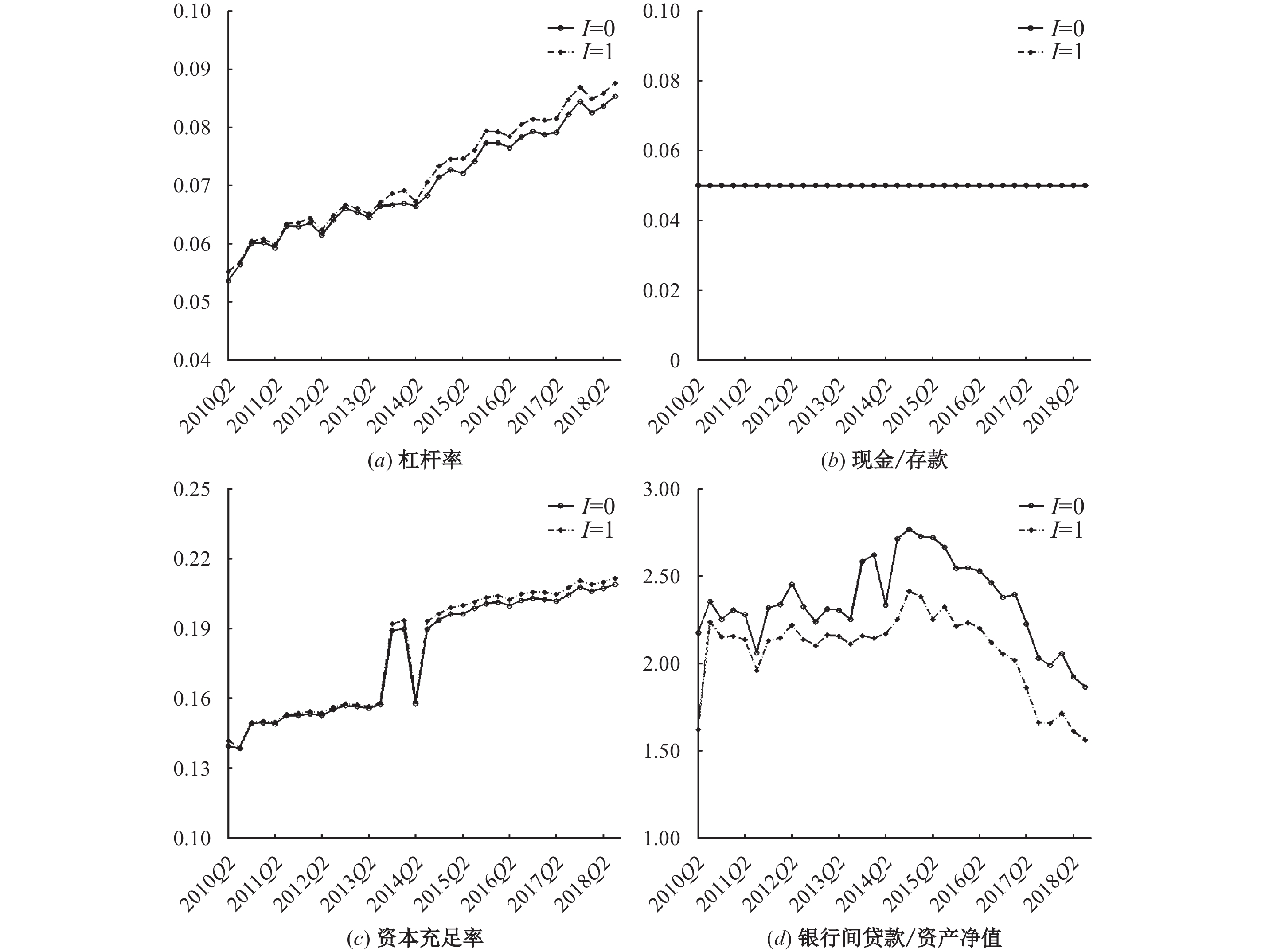图 1 杠杆率监管与无杠杆率监管下银行体系的资产配置

 Δlev levI=0 γI=0 size r cs Δlev 1.0000 −0.2248*** −0.0037 0.0957** 0.0401 0.0543 （1.0000） （0.0000） （0.3893） （0.0256） （0.3501） （0.2060） levI=0 −0.2248*** 1.0000 0.8582*** −0.1707*** −0.0925** −0.7928*** （0.0000） （1.0000） （0.0000） （0.0001） （0.0311） （0.0000） γI=0 −0.0037 0.8582*** 1.0000 −0.3804*** −0.0820** −0.6811*** （0.3893） （0.0000） （1.0000） （0.0000） （0.0559） （0.0000） size 0.0957** −0.1707*** −0.3804*** 1.0000 0.0211 0.0019 （0.0256） （0.0001） （0.0000） （1.0000） （0.6230） （0.9640） r 0.0401 −0.0925** −0.0820** 0.0211 1.0000 0.0632 （0.3501） （0.0311） （0.0559） （0.6230） （1.0000） （0.1410） cs 0.0543 −0.7928*** −0.6811*** 0.0019 0.0632 1.0000 （0.2060） （0.0000） （0.0000） （0.9640） （0.1410） （1.0000） 注：***和**分别表示在1%和5%的水平上显著，括号内为p值。

（三）杠杆率监管对危机时期风险传染的影响图 2 杠杆率监管与无杠杆率监管下的风险传染状况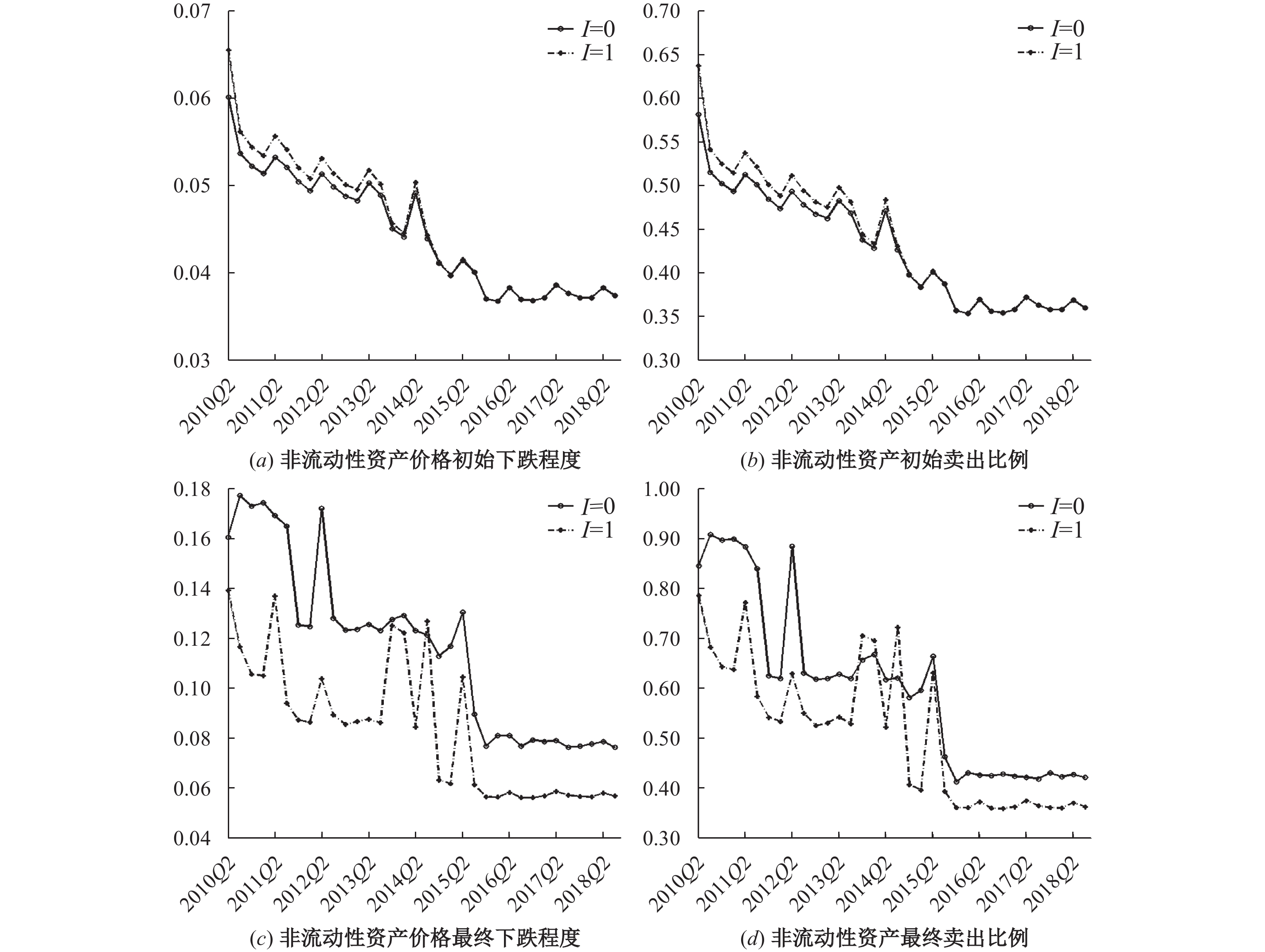图 3 金融体系非流动性资产的变化情况

（四）杠杆率监管对系统性风险的综合影响效应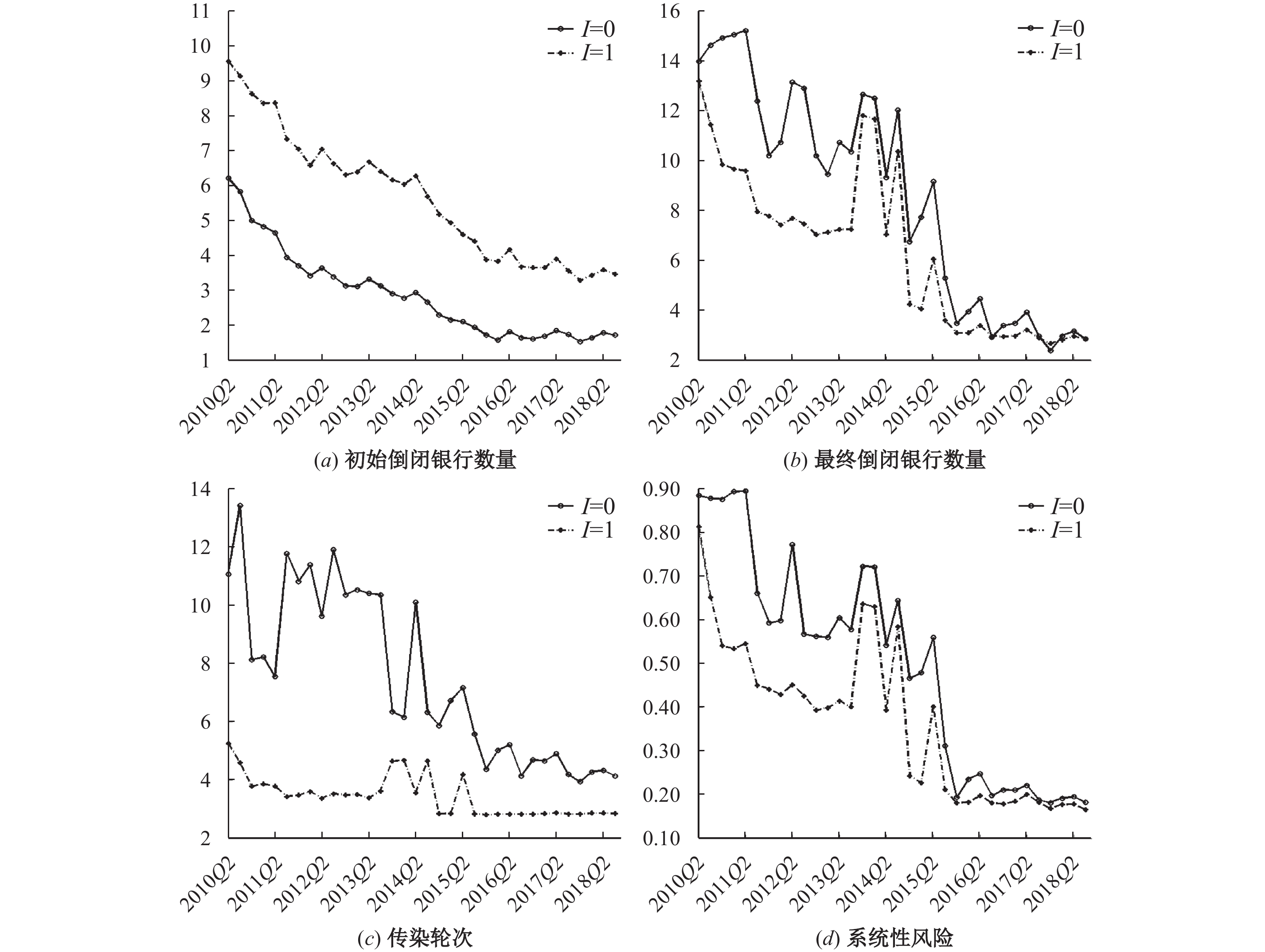图 4 杠杆率监管对系统性风险的综合影响效应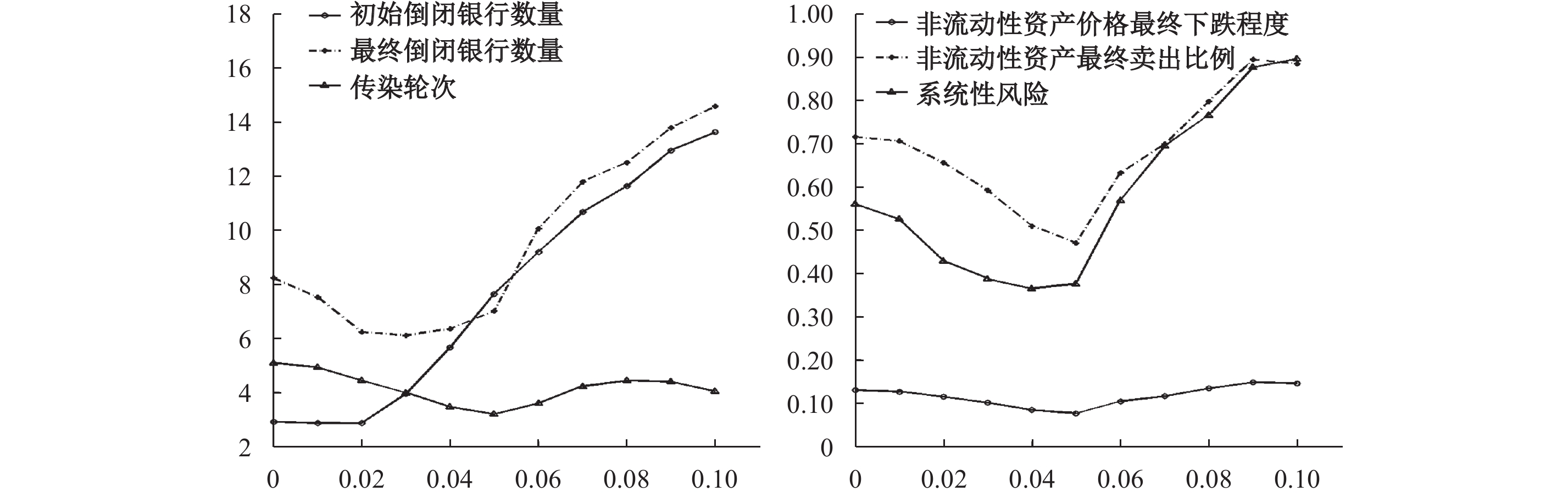图 5 不同杠杆率约束水平下的风险传染状况

（五）进一步分析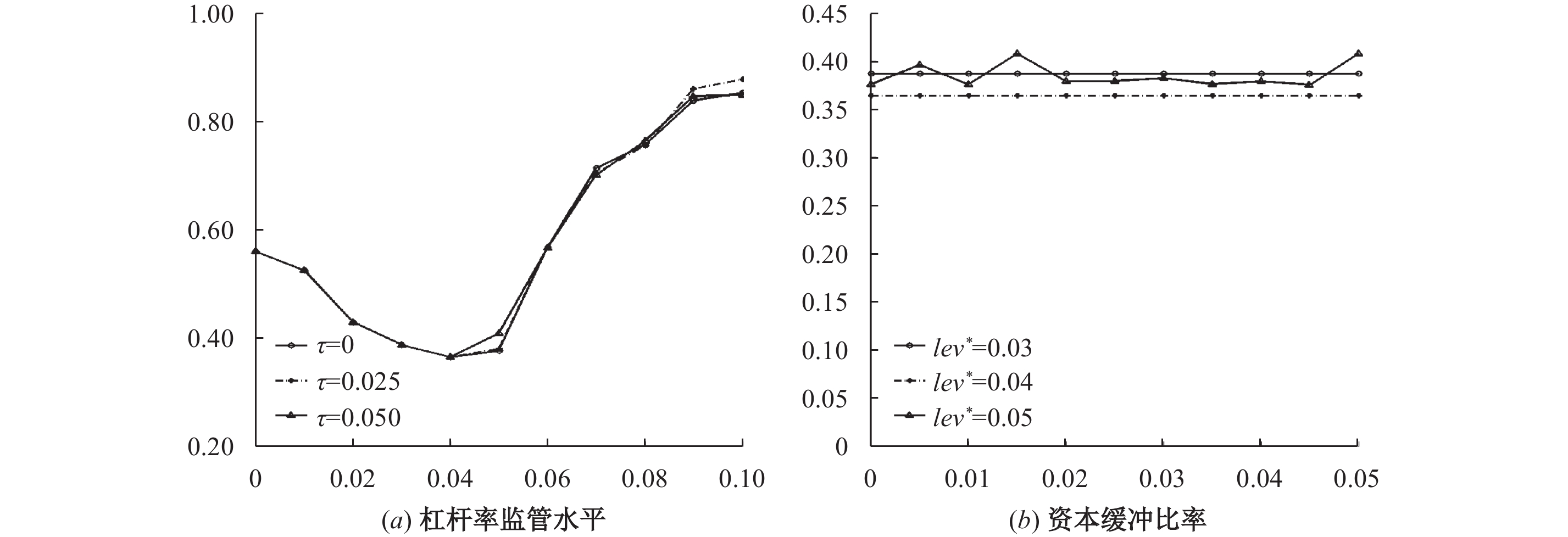图 6 资本缓冲比率对系统性风险的影响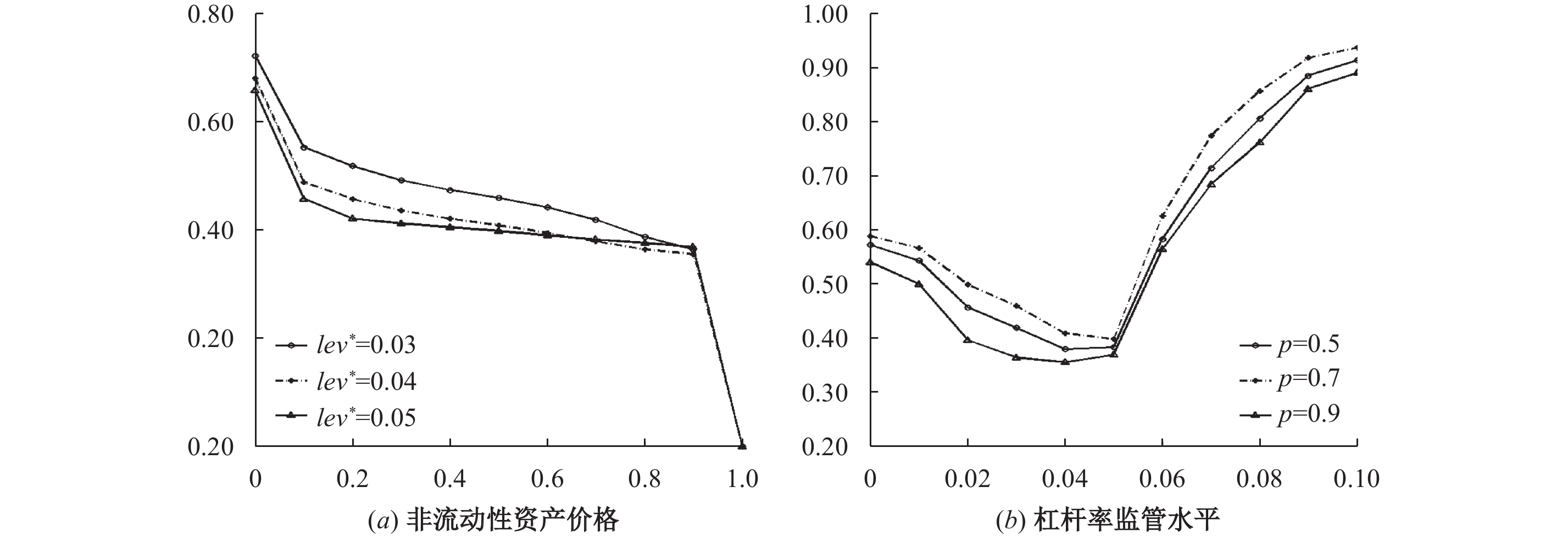图 7 价格参数对系统性风险的影响图 8 贷款回收比例对系统性风险的影响

① 本文中的非流动性资产是剔除外部贷款后的非流动性资产的统称。

② 上述项目被设置为外生变量的原因在于，银行对这些项目的支配性较弱，如外部贷款主要取决于贷款人的需求，存款取决于存款人的储蓄需求。而对于非流动性资产和银行间存贷款，银行则可以基于风险和收益来决定其最优数量，因此它们属于内生决策变量。

③ 资产负债表中的外生项目对银行来说是已发生或确定的，因此目标函数中不需要考虑其成本或收益。

④ 在不存在卖空机制的情况下，银行的非流动性资产需要大于等于0。

⑤ 金融体系稳定状态的定义请见Bluhm等（2014）。

⑥ 16家上市银行包括平安银行、宁波银行、浦发银行、华夏银行、中国民生银行、招商银行、南京银行、兴业银行、北京银行、中国农业银行、交通银行、中国工商银行、光大银行、中国建设银行、中国银行和中信银行。

⑦ 在分析引入杠杆率监管对金融体系的影响时，本文控制了模型的其他输入变量，这意味着I=0和I=1两种情况下相关指标的变化仅由杠杆率监管所引起。因此，根据表3中Δlev与银行其他指标的相关性，我们可以判断引入杠杆率监管对哪类银行杠杆率的影响更大。

⑧ 从图1中可以看出，在实现最优资产配置时，银行体系的资本充足率大致在14%以上。这意味着绝大多数银行的资本充足率远高于法定资本充足率加上缓冲资本比率的要求。

  方意. 宏观审慎政策有效性研究[J]. 世界经济, 2016(8): 25–49.  黄聪, 贾彦东. 金融网络视角下的宏观审慎管理——基于银行间支付结算数据的实证分析[J]. 金融研究, 2010(4): 1–14.  李守伟, 马钱挺, 隋新, 等. 企业信用内生网络模型及其演化研究[J]. 中国管理科学, 2019(2): 53–60.  梁琪, 李政, 卜林. 中国宏观审慎政策工具有效性研究[J]. 经济科学, 2015(2): 5–17.  马君潞, 范小云, 曹元涛. 中国银行间市场双边传染的风险估测及其系统性特征分析[J]. 经济研究, 2007(1): 68–78.  唐振鹏, 谢智超, 冉梦, 等. 网络视角下我国上市银行间市场系统性风险实证研究[J]. 中国管理科学, 2016(S1): 489–494.  王连军. 去杠杆化与银行体系稳定性研究——基于中国银行业的实证[J]. 国际金融研究, 2018(10): 55–64.  朱波, 卢露. 不同货币政策工具对系统性金融风险的影响研究[J]. 数量经济技术经济研究, 2016(1): 58–74.  Akinci O, Olmstead-Rumsey J. How effective are macroprudential policies? An empirical investigation[J]. Journal of Financial Intermediation, 2018, 33: 33–57. DOI:10.1016/j.jfi.2017.04.001  Aldasoro I, Gatti D D, Faia E. Bank networks: Contagion, systemic risk and prudential policy[J]. Journal of Economic Behavior & Organization, 2017, 142: 164–188.  Allahrakha M, Cetina J, Munyan B. Do higher capital standards always reduce bank risk? The impact of the Basel leverage ratio on the U.S. triparty repo market[[J]. Journal of Financial Intermediation, 2018, 34: 3–16. DOI:10.1016/j.jfi.2018.01.008  Bluhm M, Faia E, Krahnen J P. Monetary policy implementation in an interbank network: Effects on systemic risk[R]. SAFE Working Paper Series 46, 2014.  Bluhm M, Krahnen J P. Systemic risk in an interconnected banking system with endogenous asset markets[J]. Journal of Financial Stability, 2014, 13: 75–94. DOI:10.1016/j.jfs.2014.04.002  Blum J M. Why ‘Basel II’ may need a leverage ratio restriction[J]. Journal of Banking & Finance, 2008, 32(8): 1699–1707.  Cifuentes R, Ferrucci G, Shin H S. Liquidity risk and contagion[J]. Journal of the European Economic Association, 2005, 3(2−3): 556–566. DOI:10.1162/jeea.2005.3.2-3.556  Eisenberg L, Noe T H. Systemic risk in financial systems[J]. Management Science, 2001, 47(2): 236–249. DOI:10.1287/mnsc.47.2.236.9835  Haᴌaj G, Kok C. Modelling the emergence of the interbank networks[J]. Quantitative Finance, 2015, 15(4): 653–671. DOI:10.1080/14697688.2014.968357  Kellermann K, Schlag C. Occupy risk weighting: How the minimum leverage ratio dominates capital requirements: A Swiss example[J]. Journal of Financial Regulation and Compliance, 2013, 21(4): 353–372. DOI:10.1108/JFRC-06-2012-0019  Kiema I, Jokivuolle E. Does a leverage ratio requirement increase bank stability?[J]. Journal of Banking & Finance, 2014, 39: 240–254.  Li S W, Sui X. Contagion risk in endogenous financial networks[J]. Chaos, Solitons & Fractals, 2016, 91: 591–597.  Mirza A, Strobel F. Leverage requirements and systemic risk[R]. Working Paper, 2013.  Smith J A, Grill M, Lang J H. The leverage ratio, risk-taking and bank stability[EB/OL]. https://pdfs.semanticscholar.org/4204/7966c824378f78ab012e92e98a051ca8443d.pdf, 2017.